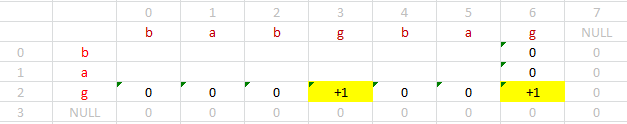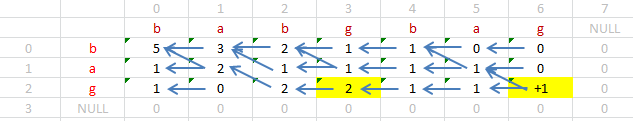## Tuesday, November 27, 2012

### Distinct Subsequences

http://www.leetcode.com/onlinejudge

Given a string S and a string T, count the number of distinct subsequences of T in S.
A subsequence of a string is a new string which is formed from the original string by deleting some (can be none) of the characters without disturbing the relative positions of the remaining characters. (ie, `"ACE"` is a subsequence of `"ABCDE"`while `"AEC"` is not).
Here is an example:
S = `"rabbbit"`, T = `"rabbit"`
Return `3`.

First approach is to use dynamic programming. Basically 2 cases:
1.  If current characters do not match, increment on S;
2.  If match, need to process two different cases:
A: Using current match, increment on both S and T
B:  keep doing case 1

```/**Small test passed, but time limit exceeded for large set
*/
class Solution {
private:
int count(string const& s, size_t s_index, string const& w, size_t w_index){
if (s_index >= s.size() || w_index >= w.size()) return 0;
if (s[s_index] != w[w_index]) return count(s,s_index+1,w,w_index);
size_t result = 0;
if (w_index +1 == w.size()) ++result;
result += count(s, s_index+1, w, w_index+1);//search the rest part based on existing findings
return result;
}//count()

public:
int numDistinct(string S, string T) {
// Start typing your C/C++ solution below
// DO NOT write int main() function
return count(S,0,T,0);
}
};```

However, due to duplicated work, this algorithm is not efficient enough to pass large data set. Similar to the hint from the longest common sequence,  a bottom-up built table can be derived.

Notice that the current index pair (tt, ss) can depend on (ss, tt+1) and/or (ss+1,tt+1), provided that if the current character on (tt,ss) match. So the algorithm is simplified to
v[tt,ss]= {v[tt,ss] + v[tt,ss+1], if no match} or {v[tt,ss]+v[tt,ss+1]+v[tt+1,ss+1], otherwise}

And the boundary condition is filling with zeros, except for those tail match of the last char in T, which are assigned to +1.

For example, T={bag}, S={babgbag}, which should return 5.And the dependencies are labeled by arrows:The complete code:

```/*passed both small and large tests**/

class Solution {
public:
int numDistinct(string S, string T) {
// Start typing your C/C++ solution below
// DO NOT write int main() function
vector<int> w(S.size()+1, 0);
vector<vector<int> > v(T.size()+1, w);
int maxS = S.size()-1;
int maxT = T.size()-1;
for(int ii = 0; ii < S.size(); ++ii){
if (T[maxT] == S[ii]) v[maxT][ii] = 1;
}//init
for(int ss = maxS-1; ss>=0; --ss){
for(int tt = maxT; tt >= 0; --tt){
v[tt][ss] += v[tt][ss+1];
if (T[tt] == S[ss]) v[tt][ss] += v[tt+1][ss+1] ;
}
}
return v;
}
};```Anonymous said...

subsequences of bag are - 8 and all are present in S , so why is answer 5 ?

LN said...

babgbag:
ba*g***
ba****g
b****ag
**b**ag
****bag

Unknown said...

can you help me to understand problem.
S = "rabbbit", T = "rabbit"
T has many distinct subsequence Eg. rabbit, rab, rait , rabit, ait, bit, ans many more
ans all of them present in S.
Then how the answer is 3.